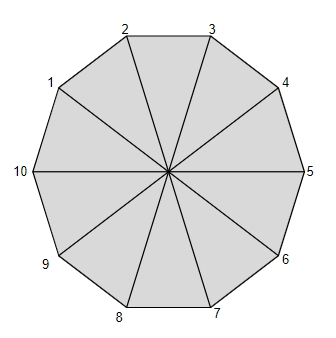# Program to Calculate the Perimeter of a Decagon in C program

CServer Side ProgrammingProgramming

## What is Decagon?

Given with side, the task is to calculate the perimeter of decagon. Decagon is a type of polygon with 10-sides that’s why it is also known as 10-gon polygon. It have 10 vertices and edges. A regular decagon has sides of equal length and each internal angle of degree 144.

Given below is the figure of DecagonTo calculate the volume and surface area of Frustum of cone there is a formula

Perimeter = 10 * Side

## Example

Input-: side=10
Output-: perimeter of Decagon is : 100

Input -: side = 20
Output -: perimeter of Decagon is : 200

## Algorithm

Start
Step 1 -> declare function for finding the perimeter
void perimeter(int n)
declare variable as int perimeter
set perimeter=10*n
print perimeter
step 2 -> In main()
declare int n=10
perimeter(n)
Stop

## Example

#include <stdio.h>
// Function for finding the perimeter
void perimeter(int n){
int perimeter;
perimeter = 10 * n;
printf("perimeter of Decagon is : %d", perimeter);
}
int main(){
int n=10;
perimeter(n);
return 0;
}

## Output

perimeter of Decagon is : 100